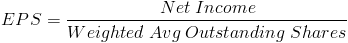# Earnings Per Share

EPS Calculator (Click Here or Scroll Down)The formula for earnings per share, or EPS, is a company's net income expressed on a per share basis. Net income for a particular company can be found on its income statement. It is important to note that the earnings per share formula only references common stock and any preferred stock dividends is subtracted from the net income, if applicable.

## Per Share

The denominator of the earnings per share is the weighted average of outstanding shares of common stock. When the amount of common shares changes mid-year, the "per share" portion requires additional calculation. The per share portion is weighted based on the length of time each number of shares is in effect.

An example of the weighted average would be a company who has 100,000 outstanding common shares for 9 months and due to issuing new common stocks, has 120,000 outstanding shares for the remaining 3 months. The weight for 100,000 shares would be 9/12(.75) and the weight for 120,000 shares would be 3/12(.25).

To calculate the weighted average from the example:
(.75)100,000 + (.25)120,000 = 75,000 + 30,000 = 105,000

## In Relation to Dividends

Earnings and dividends are not one in the same. Companies, many times, retain some of their earnings for future growth. The amount of earnings that are paid out in dividends to common stockholders can be found from using the dividend payout ratio formula. A company that pays 20% of their net income to common stockholders will have a 20% payout ratio.

A company that pays all their earnings out to common stockholders will have a 100% payout ratio. Stocks that pay out all of their earnings to common stockholders are considered zero growth stocks, as none of the earnings the company receives is retained for future growth. In these circumstances, earnings will equal dividends. Likewise, earnings per share will be equal to dividends per share.

## Use of Earnings Per Share Formula

The earnings per share formula is used in other formulas such as the P/E ratio formula and, on occasion, stock valuation.

New to Finance?